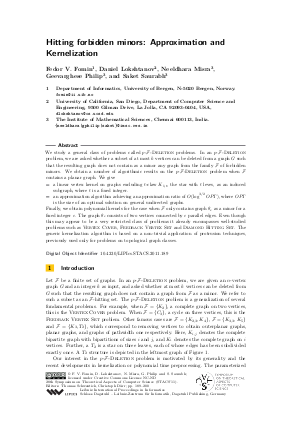Document# Hitting forbidden minors: Approximation and Kernelization

### Authors Fedor V. Fomin, Daniel Lokshtanov, Neeldhara Misra, Geevarghese Philip, Saket Saurabh## File

LIPIcs.STACS.2011.189.pdf
• Filesize: 2.96 MB
• 12 pages

## Cite As

Fedor V. Fomin, Daniel Lokshtanov, Neeldhara Misra, Geevarghese Philip, and Saket Saurabh. Hitting forbidden minors: Approximation and Kernelization. In 28th International Symposium on Theoretical Aspects of Computer Science (STACS 2011). Leibniz International Proceedings in Informatics (LIPIcs), Volume 9, pp. 189-200, Schloss Dagstuhl - Leibniz-Zentrum für Informatik (2011)
https://doi.org/10.4230/LIPIcs.STACS.2011.189

## Abstract

We study a general class of problems called F-Deletion problems. In an F-Deletion problem, we are asked whether a subset of at most k vertices can be deleted from a graph G such that the resulting graph does not contain as a minor any graph from the family F of forbidden minors. We obtain a number of algorithmic results on the F-Deletion problem when F contains a planar graph. We give - a linear vertex kernel on graphs excluding t-claw K_(1,t), the star with t leaves, as an induced subgraph, where t is a fixed integer. - an approximation algorithm achieving an approximation ratio of O(log^(3/2) OPT), where \$OPT\$ is the size of an optimal solution on general undirected graphs. Finally, we obtain polynomial kernels for the case when F only contains graph theta_c as a minor for a fixed integer c. The graph theta_c consists of two vertices connected by \$c\$ parallel edges. Even though this may appear to be a very restricted class of problems it already encompasses well-studied problems such as Vertex Cover, Feedback Vertex Set and Diamond Hitting Set. The generic kernelization algorithm is based on a non-trivial application of protrusion techniques, previously used only for problems on topological graph classes.
##### Keywords
• kernelization

## Metrics

• Access Statistics
• Total Accesses (updated on a weekly basis)
0